Next: Expectation Values Up: Fundamental Concepts Previous: Observables

# Measurements

We have seen that a measurement of some observable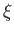of a microscopic system causes the system to jump into one of the eigenstates of. The result of the measurement is the associated eigenvalue (or some function of this quantity). It is impossible to determine into which eigenstate a given system will jump, but it is possible to predict the probability of such a transition. So, what is the probability that a system in some initial state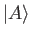makes a transition to an eigenstate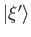of an observable, as a result of a measurement made on the system? Let us start with the simplest case. If the system is initially in an eigenstatethen the transition probability to a eigenstate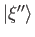corresponding to a different eigenvalue is zero, and the transition probability to the same eigenstateis unity. It is convenient to normalize our eigenkets such that they all have unit norms. It follows from the orthogonality property of the eigenkets that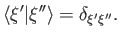(1.53)

For the moment, we are assuming that the eigenvalues ofare all different.

Note that the probability of a transition from an initial eigenstateto a final eigenstateis the same as the value of the inner product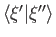. Can we use this correspondence to obtain a general rule for calculating transition probabilities? Well, suppose that the system is initially in a (normalized) statewhich is not an eigenstate of. Can we identify the transition probability to a final eigenstatewith the inner product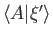? Unfortunately, this is not possible becauseis, in general, a complex number, and complex probabilities do not make any sense. Let us try again. Suppose that we identify the transition probability with the modulus squared of the inner product,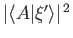? This quantity is definitely a positive number (so it could be a probability). This guess also gives the right answer for the transition probabilities between eigenstates. In fact, it is the correct guess.

Because the eigenstates of an observableform a complete set, we can express any given stateas a linear combination of them. It is easily demonstrated that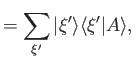(1.54)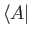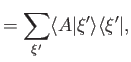(1.55)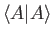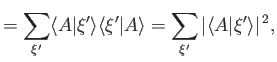(1.56)

where the summation is over all the different eigenvalues of, and use has been made of Equation (1.21), as well as the fact that the eigenstates are mutually orthogonal. Note that all of the previous results follow from the extremely useful result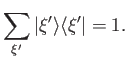(1.57)

(See Exercise 9.) The relative probability of a transition to an eigenstate, which is equivalent to the relative probability of a measurement ofyielding the result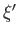, is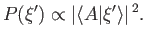(1.58)

The absolute probability is clearly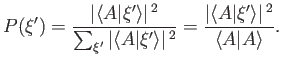(1.59)

If the ketis normalized such that its norm is unity then this probability simply reduces to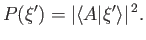(1.60)Next: Expectation Values Up: Fundamental Concepts Previous: Observables
Richard Fitzpatrick 2016-01-22